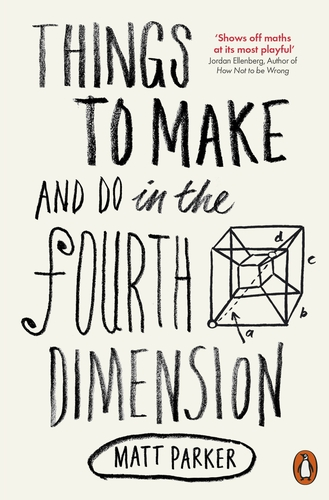# You're reading: Posts Tagged: book excerpt

### How many ways to shuffle a pack of cards?

This is an excerpt from friend of The Aperiodical, Matt Parker’s book, “Things to Make and Do in the Fourth Dimension”, which is out now in paperback.There’s a lovely function in mathematics called the factorial function, which involves multiplying the input number by every number smaller than it. For example: $\operatorname{factorial}(5) = 5 \times 4 \times 3 \times 2 \times 1 = 120$. The values of factorials get alarmingly big so, conveniently, the function is written in shorthand as an exclamation mark. So when a mathematician writes things like $5! = 120$ and $13! = 6,\!227,\!020,\!800$ the exclamation mark represents both factorial and pure excitement. Factorials are mathematically interesting for several reasons, possibly the most common being that they represent the ways objects can be shuffled. If you have thirteen cards to shuffle, then there are thirteen possible cards you could put down first. You then have the remaining twelve cards as options for the second one, eleven for the next, and so on – giving just over 6 billion possibilities for arranging a mere thirteen cards.